# Leetcode遇上C++（一）

## 前言：

为了锻炼自己的编程能力，决定尝试着刷下Leetcode题库，也为自己毕业时找软件开发岗位打下基础，当然咯，作为非科班出身的我，在编程上面还有很大的问题，甚至连最基本的知识都未掌握好，但是，我相信功夫不负有心人。通过动手编程来学习是我感觉最舒服的一种学习方式，因此，特此记录，以备将来复习。

## 一、Two Sum

### 题目如下：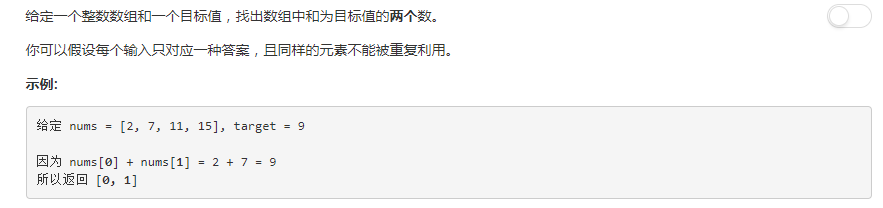### C++解答（二分查找法）：

class Solution {

public:
vector<int> twoSum(vector<int> &numbers, int target)

{
vector<int> num = numbers;
// （1）所有的容器都是类模板，要定义某种特殊的容器，必须在容器后加一对尖括号，
// 尖括号里面提供容器中存放的元素的类型。
// 所有的容器类型都定义了默认构造函数，用于创建指定类型的空容器对象，注意，默认构造函数不带参数。
// （2）容器的赋值操作：例如c1 = c2, 表示删除容器c1的所有元素，然后将c2的元素赋值给c1。
// c1和c2的类型（包括容器类型和元素类型）必须相同。

std::sort(num.begin(), num.end());
// std::sort()是一个快速排序函数，其函数声明如下:
// void sort( iterator start, iterator end );
// 其中，num.begin()返回一个迭代器，它指向容器num的第一个元素，
// num.end()返回一个迭代器，它指向容器num的最后一个元素的下一位置。

int length = (int)numbers.size();
// size操作返回容器内元素的个数，例如，c.size（）返回容器c中的元素个数，返回类型为c::size_type。
// size_type 相当于 unsigned int类型, 使用size_type 主要是为了适应不同的平台, int 类型大小会根据不同平台而不同

vector<int>::iterator prior = num.begin();
vector<int>::iterator behind = --num.end();
// vector<int>::iterator是定义向量迭代器，下面介绍一些应用于容器迭代器的关系操作符：
// 例如：>,>=,<,<=, 即当一个迭代器指向的元素在容器中位于另一个迭代器指向的元素之前，则前一个迭代器小于后一个迭代器。
// 注意： 关系操作符的两个迭代器必须指向同一个容器中的元素或者超出容器末端的下一位置。

int sum = 0;

vector<int> index;

while( prior < behind)

{
sum = *prior + *behind ;
// vector迭代器的解引用运算，使用解引用操作符（*操作符）来访问迭代器所指向的元素，解引用操作符返回迭代器当前所指向的元素的引用

if(sum == target)
{
for(int i = 0; i < length; ++i)
{
if(numbers[i] == *prior)
{
index.push_back(i);
// push_back是在容器中添加元素的一种方法，所有的顺序容器都支持push_back操作。
// 例如，c.push_back（t）,在容器c的尾部添加值为t的元素，返回void类型。
}
else if(numbers[i] == *behind)
{
index.push_back(i);
}
if(index.size() == 2)
{
break;
}
}
break;
}

else if(sum > target)
{
--behind;
}
else
{
++prior;
}
}

return index;
}

};


### 提交结果：### 题目如下：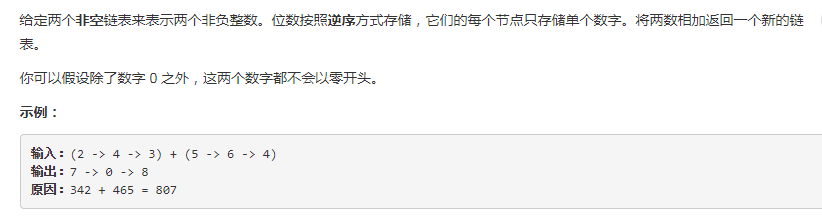### C++解答：

/**
* struct ListNode {
*     int val;
*     ListNode *next;
*     ListNode(int x) : val(x), next(NULL) {}
* };
*/

class Solution {

public:

{
ListNode* pa = l1;
ListNode* pb = l2;

int temp_value =0;
int carry_bit =0;

for ( ; pa !=NULL || pb !=NULL; )
{

if (pa !=NULL && pb !=NULL)
{
temp_value = (pa->val + pb->val + carry_bit) % 10 ;
carry_bit = (pa->val + pb->val + carry_bit) / 10 ;
latter_ListNode -> next =  new ListNode(temp_value);
pa = pa->next;
pb = pb->next;
}

else if (pa ==NULL && pb !=NULL)
{
temp_value = (0 + pb->val + carry_bit) % 10 ;
carry_bit = (0 + pb->val + carry_bit) / 10 ;
latter_ListNode -> next =  new ListNode(temp_value);
pb = pb->next;

}

else if (pa !=NULL && pb ==NULL)
{
temp_value = (pa->val + 0 + carry_bit) % 10 ;
carry_bit = (pa->val + 0 + carry_bit) / 10 ;
latter_ListNode -> next = new ListNode(temp_value);
pa = pa->next;

}

latter_ListNode =  latter_ListNode -> next;
}

if (carry_bit > 0)
{
latter_ListNode -> next =  new  ListNode(carry_bit);
}

}
};

### 提交结果：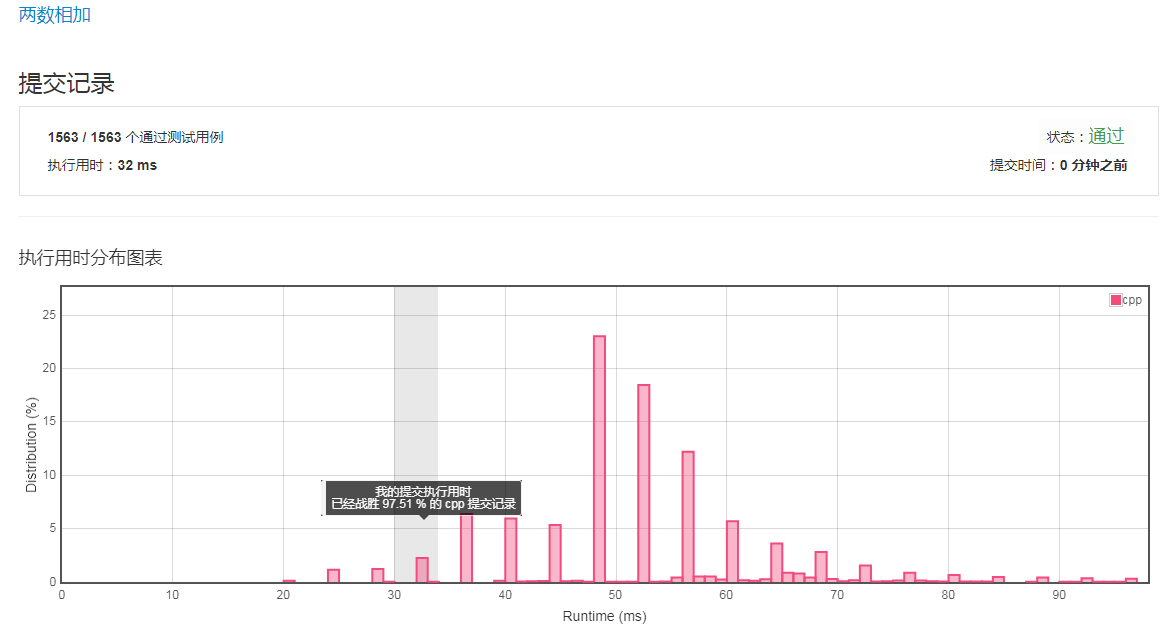## 三、Longest Substring Without Repeating Characters

### 题目如下：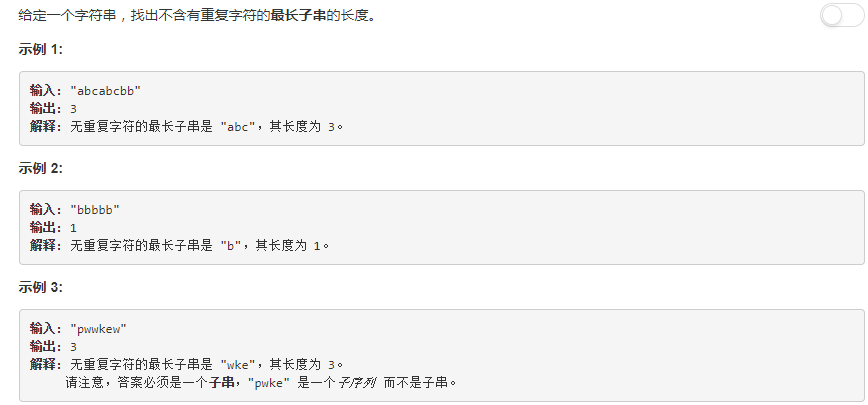### 解题思路如下：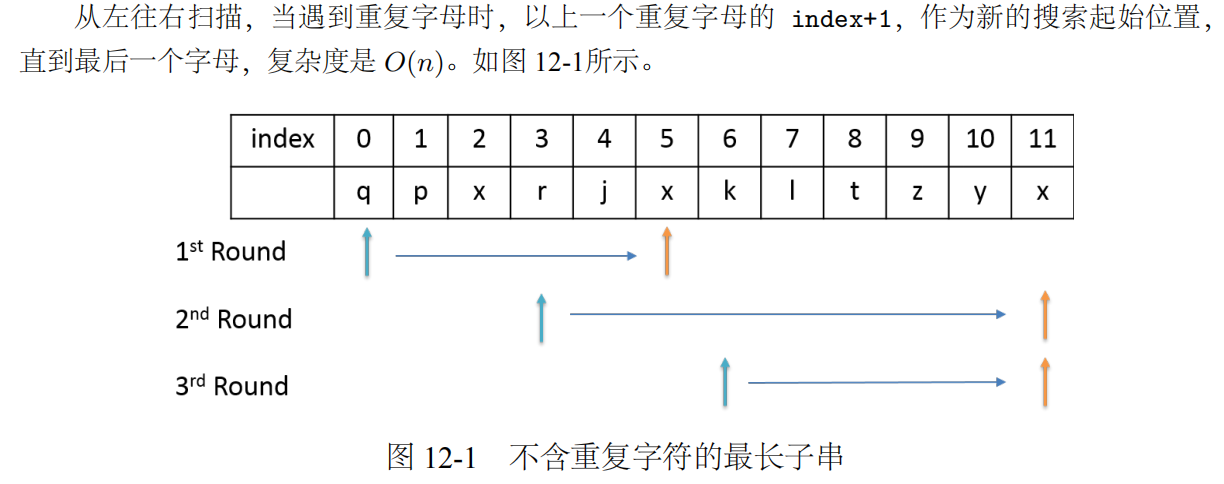### C++ 解答：

class Solution {

public:

int lengthOfLongestSubstring(string s)
{

map<char, int>  last_appear_index;
// map是键-值对的集合，map类型通常可以理解为关联数组:可使用键作为下标来获取一个值。
// 在定义map对象时必须分别指明键和值的类型，上述语句表示定义了名为 last_appear_index
// 的map对象，由string类型的键索引，关联的值则为int型。
// string类型的键为s中的元素，int型的值为最新出现的位置索引。

int substring_start = 0, longest = 0;

map<char, int>::iterator  map_it;

for (int i = 0; i < s.length(); i++)
{
map_it = last_appear_index.find(s[i]);
// map容器提供了find操作，用于检查某个键是否存在而不会插入该键，
// 例如，m.find（k）表示：如果m容器中存在按K索引的元素，则返回指向该元素的迭代器；
// 如果不存在，则返回超出末端迭代器。
// 如果希望当具有指定键的元素存在时，就获取该元素的引用，否则就不在容器中创建新的元素，那么应该使用find。

if (map_it != last_appear_index.end() && map_it->second >= substring_start)
{
substring_start = map_it->second + 1;
// map迭代器返回 value_type类型（存储元素的键以及值的pair类型）的值————包含const key_type和mapped_type 类型成员的pair对象，
// 对于pair类，可以直接访问其数据成员，其数据成员都是公有的，分别命名为first和second。
// 因此，对map迭代器进行解引用获得pair对象中，它的first成员存放键，为const，而second成员则存放值。
// 因此 substring_start 指向第一个重复元素的下一元素。
}

last_appear_index[s[i]] = i;
// 将重复元素的位置索引更新为最新出现的位置索引

longest = max(longest, i-substring_start+1);
// 更新不重复子字符串的长度

}
return longest;

}
};

### 提交结果：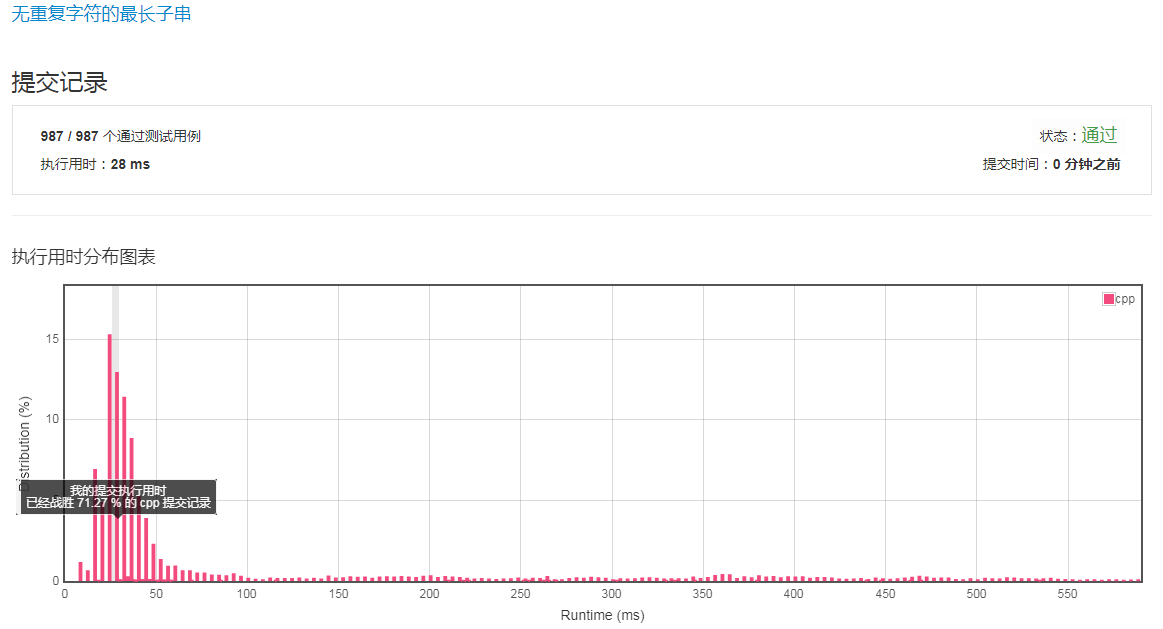## 题目如下：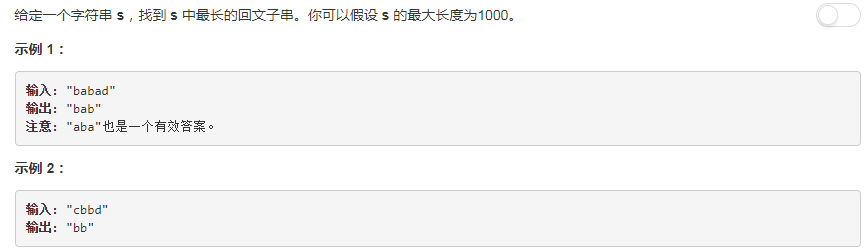## 解法一：

首先说下什么是回文串，就是正读反读都一样的字符串，比如 "bob", "level", "noon" 等等。那么最长回文子串就是在一个字符串中的那个最长的回文子串。我们知道传统的验证回文串的方法就是两个两个的对称验证是否相等，那么对于找回文字串的问题，就要以每一个字符为中心，像两边扩散来寻找回文串，这个算法的时间复杂度是O(n*n)，就是要注意奇偶情况，由于回文串的长度可奇可偶，比如"bob"是奇数形式的回文，"noon"就是偶数形式的回文，两种形式的回文都要搜索。

C++解答：

class Solution {
public:
string longestPalindrome(string s)
{

if (s.length() <= 1)
return s;
// 当字符串只有一个元素的时候，无论如何都是回文字符串。

int  start = 0;
int  max_len = 0;
//  最长回文子串的起点 ,长度

int  prior_index =0;
int  next_index = 0;

for (int i=0; i <s.length()-1; ++i)
{
if (s[i] == s[i+1])
// 相邻两个元素相同，说明它们有偶数形式的回文的可能。
{
prior_index = i;
next_index = i+1;
searchPalindrome(s, prior_index, next_index, max_len, start);
}
// 再检测该字符有奇数形式的回文的可能。
prior_index = i;
next_index = i;
searchPalindrome(s, prior_index, next_index, max_len, start);
}
return s.substr(start , max_len);
// substr函数，返回当前string对象的子串，例如 s.substr（pos，n）
// 返回一个string类型的字符串，它包含s中从下标pos开始的n个字符。
}

void  searchPalindrome(string s, int prior_index, int next_index, int &max_len, int &start)
{
int step = 0;

while( prior_index-step-1 >=0 && next_index+step+1 < s.length())
//  当往左右两边扩张而不越界时。
{
if(s[prior_index - step - 1] != s[next_index + step + 1] )
break;
++step;
}

int  width = next_index - prior_index + 1 + 2*step;
if (max_len < width)
{
max_len = width;
start = prior_index - step;
}
// 记录此次检测的回文的长度，然后与先前的历史最长回文的长度相比较，
// 如果相等我们就不管了，我们只记录最先出现的等长回文字符串；
// 如果小于，就更新回文长度以及最长回文字符串的起点下标。
}

};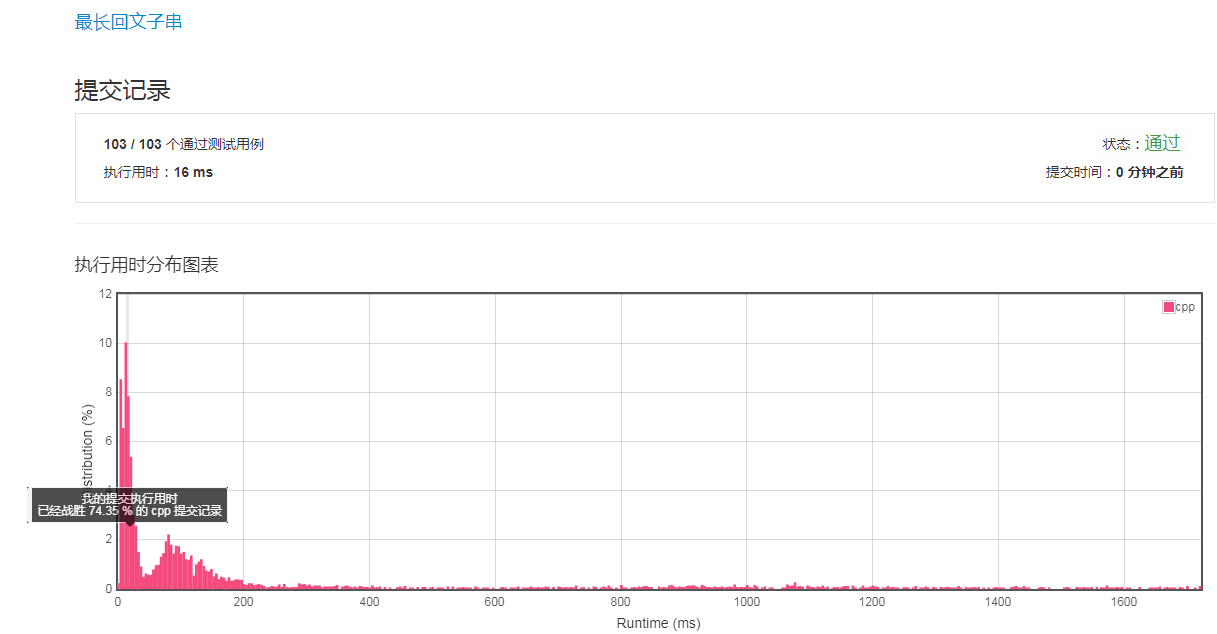## 解法二：

此题还可以用动态规划（Dynamic Programming）来解，首先我们创建一个二维数组dp，其中dp[j] [i]表示字符串区间[j, i]是否为回文串，当j = i时，只有一个字符，肯定是回文串，如果 i= j + 1，说明是相邻字符，此时需要判断s[i]是否等于s[j]，如果 i 和 j 不相邻，即 i - j >= 2时，除了判断s[i] 和 s[j] 相等之外，还要判断 dp[j + 1] [i - 1]，若为真，就是回文串。

C++ 解答：

class Solution {

public:

string longestPalindrome(string s)

{
if(s.size() <= 1)
return s;

int dp[s.size()][s.size()] = {0};
int max_len = 1, start = 0;           // 最长回文子串的长度，起点

for (int i = 0; i < s.size(); ++i)
{
for (int j = 0; j < i; ++j)
{
dp[j][i] = (s[i] == s[j] && (i - j < 2 || dp[j + 1][i - 1]));
if (dp[j][i] && max_len < i - j + 1)
{
max_len = i - j + 1;
start = j;
}
}
dp[i][i] = 1;
}

return s.substr(start, max_len);
}

};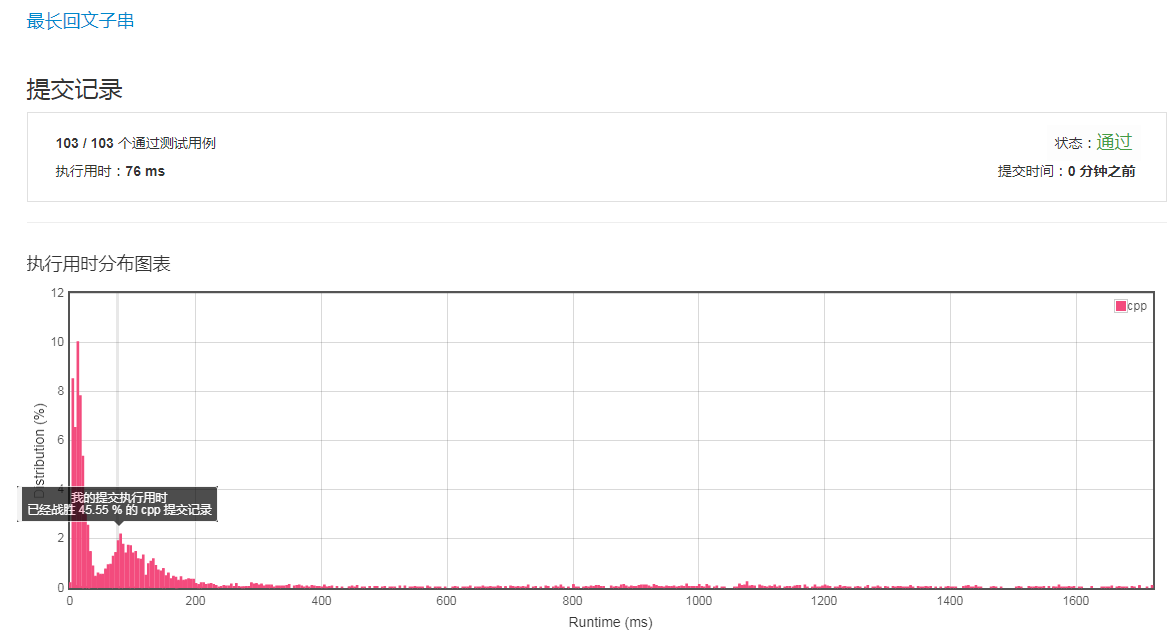## 五、Reverse Integer

### 题目如下：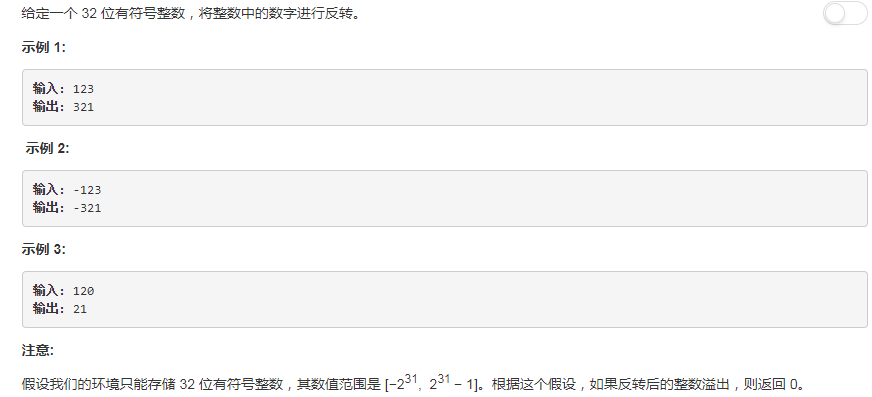## C++解答：

class Solution {

public:

int reverse(int x)
{
int res = 0;
int temp = 0;

while(x !=0 )
{
temp = res * 10 + x % 10 ;

if ( temp / 10 != res)
return 0;
// 这个if 语句用来检查是否发生溢出，
// 如果发生溢出，返回 0 结束循环。

res = temp;
x = x / 10;
}

return res;
}

};

// 这个地方为什么不分开考虑正数和负数的情况呢？这是因为正负号并不影响计算。
// 首先我们来回顾下，C++中的取整和取模运算：
// （1）除法的取整分为三类：向上取整、向下取整、向零取整，而C/C++采用向零取整方式。
//    所谓向零取整，指向0方向取最接近精确值的整数，换言之就是舍去小数部分，因此又称截断取整。
//    在这种取整方式下，7/4=1，7/(-4)=-1，6/3=2，6/(-3)=-2。
// （2）负数取模呢？ 对C/C++而言，解决负数取模问题的关键是公式：余数=被除数-商×除数。
// 因此由C/C++向零取整的整除方式可知，7%(-4)=3，(-7)%4=-3，(-7)%(-4)=-3。

// 理解了上面的知识以后，我们来演示一个简单的例子，将负数 -256进行反转：
// 第一次循环： res = 0 * 10 + （-256） % 10 = 0 + （-6）= -6，  x = （-256） / 10 = -25；
// 第二次循环： res = -6 * 10 + （-25） % 10 = -60 + （-5）= -65，  x = （-25） / 10 = -2；
// 第三次循环： res = -65 * 10 + （-2） % 10 = -650 + （-2）= -652，  x = （-2） / 10 = 0；
// 退出循环，返回结果 -652， 显然负号并不影响计算过程。

## 提交结果：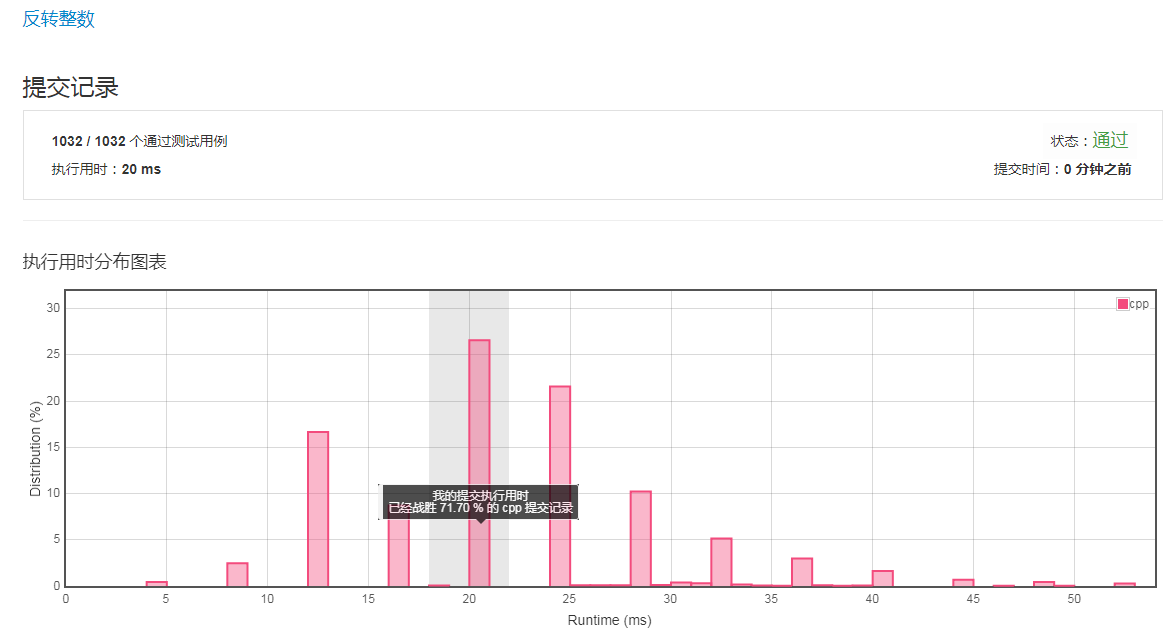## 题目如下：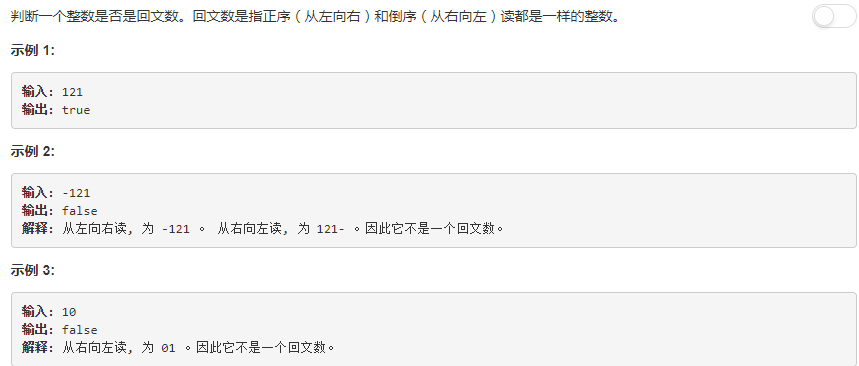## 解法一（反转全部数字）：

C++解答：

class Solution {

public:

bool isPalindrome(int x)
{
if ( x < 0 || (x != 0 && x % 10 ==0))
return false;
// 显然，整数的最低位也不能为0，例如 10 就不是回文数，
// 因此除数字 0 以外，所以如果发现某个正数的末尾是 0 了，就可以直接排除了。
// 此外，负数也一定不是回文数。

int res = 0;
int x_copy = x;

while ( x!=0 )
{

if (res > INT_MAX/10 || (res == INT_MAX/10 && x % 10 >7) )
return false;
// 如果是回文数，反转后仍是原数字，就不可能溢出，只要溢出一定不是回文数

res = res * 10 + x % 10;
x = x / 10 ;
}

return x_copy == res;
}

};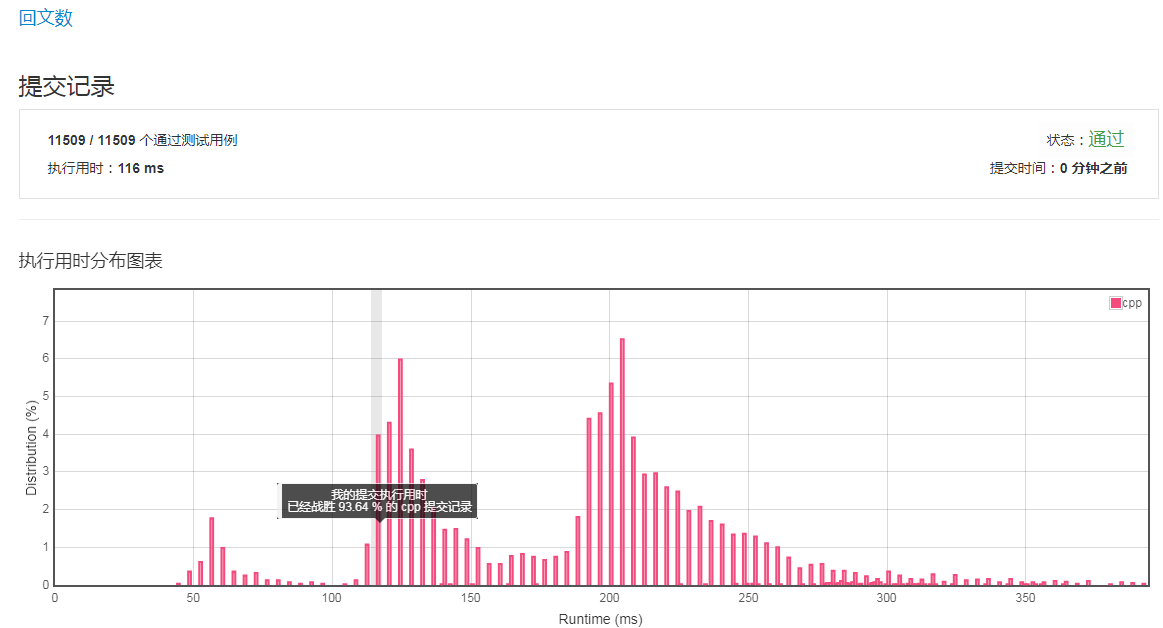## 解法二（反转一半数字）：

具体做法是，每次通过对10取余，取出最低位的数字，然后加到取出数的末尾，就是将 revertNum 乘以10，再加上这个余数，这样我们的翻转也就同时完成了，每取一个最低位数字，x都要自除以10。这样当 revertNum大于等于x的时候循环停止。由于回文数的位数可奇可偶，如果是偶数的话，那么revertNum 就应该和x相等了；如果是奇数的话，那么最中间的数字就在revertNum 的最低位上了，我们除以10以后应该和x是相等的。

C++ 解答：

class Solution {

public:

bool isPalindrome(int x)
{
if (x < 0 || (x % 10 == 0 && x != 0))
return false;
// 显然，当 x < 0 时，x 不是回文数。
// 同样地，除 0 以外，如果数字的最后一位是 0，则可以直接排除

int revertNum = 0;

while (x > revertNum)
{
revertNum = revertNum * 10 + x % 10;
x /= 10;
}

return x == revertNum || x == revertNum / 10;
// 当奇回文数时，我们可以通过 revertedNumber/10 去除处于中位的数字。
// 例如，当输入为 12321 时，在 while 循环的末尾我们可以得到 x = 12，revertedNumber = 123，
// 由于处于中位的数字不影响回文（它总是与自己相等），所以我们可以简单地将其去除。
}

};## 七、Remove Duplicates from Sorted Array(26)

### 题目如下：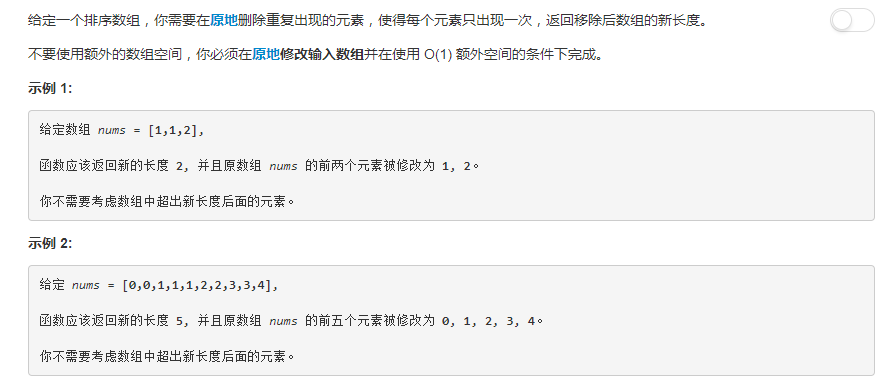### C++解题：

class Solution {

public:
int removeDuplicates(vector<int>& nums)
{
// 考虑空向量的情况
if (nums.size() == 0)

return 0;

int i = 0;

for (int j=1; j < nums.size();j++)
{
if (nums[j] != nums[i])
{
i++;

nums[i] = nums[j];
}
}

return i+1;
}
};

### 提交结果：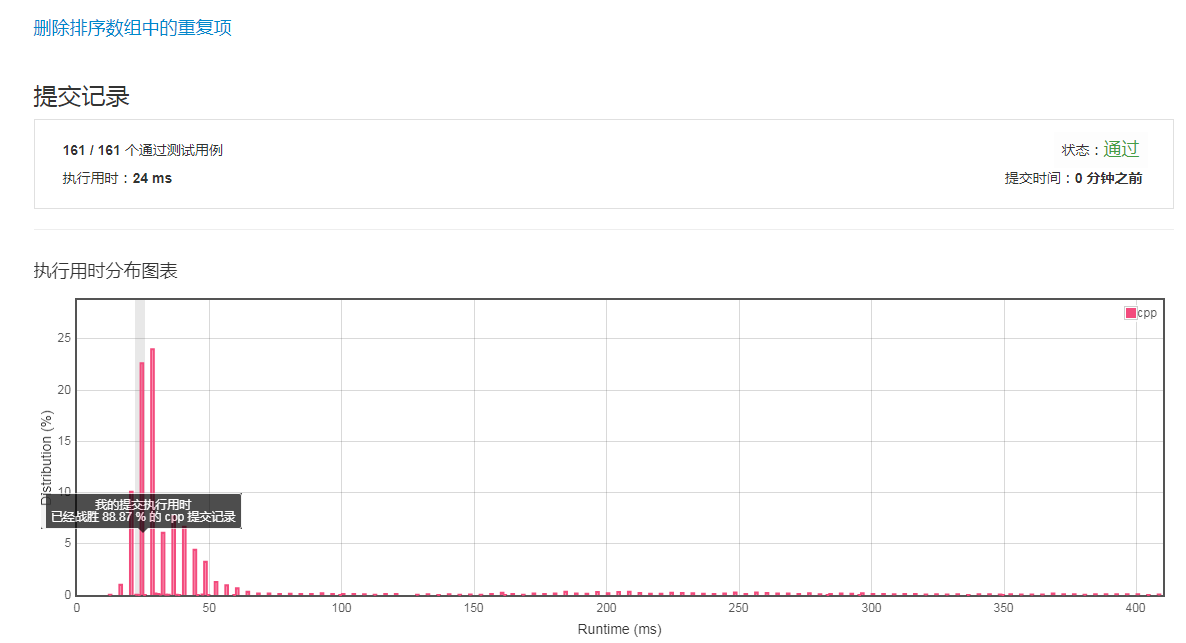## 八、Power of Two (231)

### 解题思路：

通过对1，2，8，16这四个数字分析，发现这些数字的二进制形式分别为：1，10，100，1000。从二进制的表示可以看出，如果一个数是2的n次方，那么这个数对应的二进制表示中只有一位是1，其余位都为0，因此，判断一个数是否为2的n次方可以转换为这个数对应的二进制表示中是否只有一位为1。如果一个数的二进制表示只有一个位为1，例如num=00010000，那么num-1的二进制表示为：num-1=00001111，由于num与num-1二进制表示中每一位都不相同，因此num&(num-1)的运算结果为0，可以利用这个方法来判断一个数是否为2的n次方。

### C++解答：

class Solution {

public:
bool isPowerOfTwo(int n)
{
if (n < 1)
return false;

if ( n != 1 && n % 2 !=0)
return false;

return int(n & (n-1))== 0;
}
};

### 提交结果：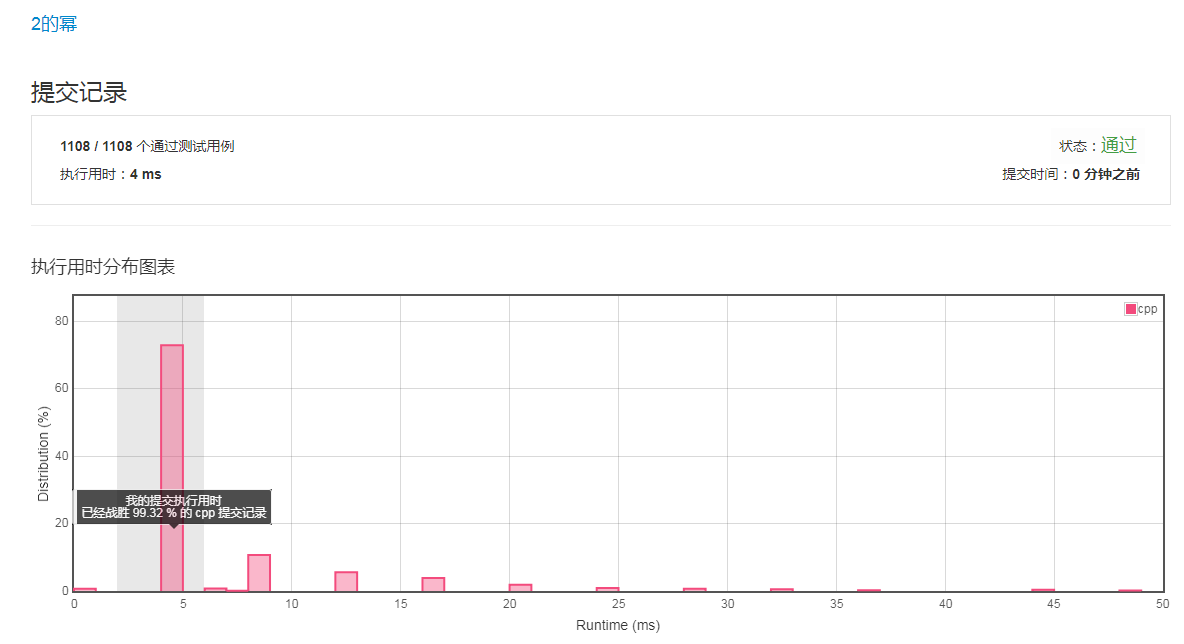## 九、 830. Positions of Large Groups

### 题目如下：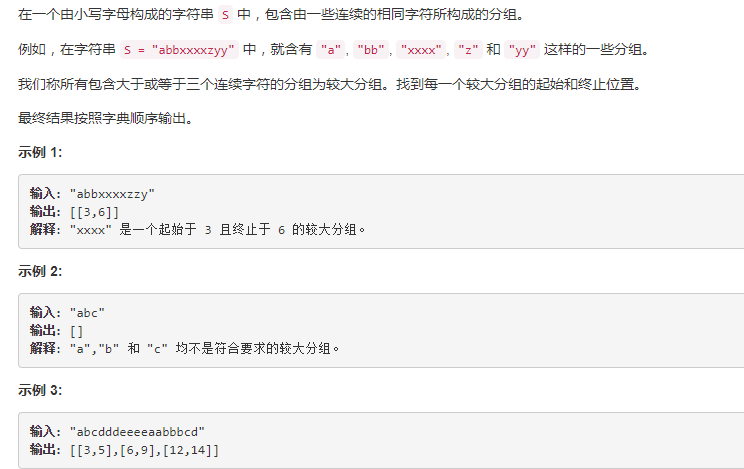### 解法（一）：

class Solution{

public:

vector<vector<int>> largeGroupPositions(string S)
{
int left = 0;

vector<vector<int>> result;

for (int right=1; right<S.length();++right)
{

if (S[right] !=S[left])
left= right ;

else
{

if ( (right < S.length()-1 &&  S[right+1] != S[right] && (right-left)>=2) ||  (right ==S.length()-1 && (right-left)>=2) )
{
result.push_back({left,right});
left = right ;
}
}

}

return  result;
}
};

### 解法（二）：

class Solution{

public:

vector<vector<int>> largeGroupPositions(string S)

{

vector<vector<int>> ret;

for(int i=0;i<S.size();)

{

int temp=S[i],j=i+1;

while(S[j]==temp && j<S.size())
j++;

if(j-i>=3)
ret.push_back({i,j-1});
// 这种用法相当于：
//  vector<int> temp;
//  temp.push_back(i);
//  temp.push_back(j);
//  ret.push_back(temp);
//  需要掌握。

i=j;

}

return ret;

}

};

### 提交结果：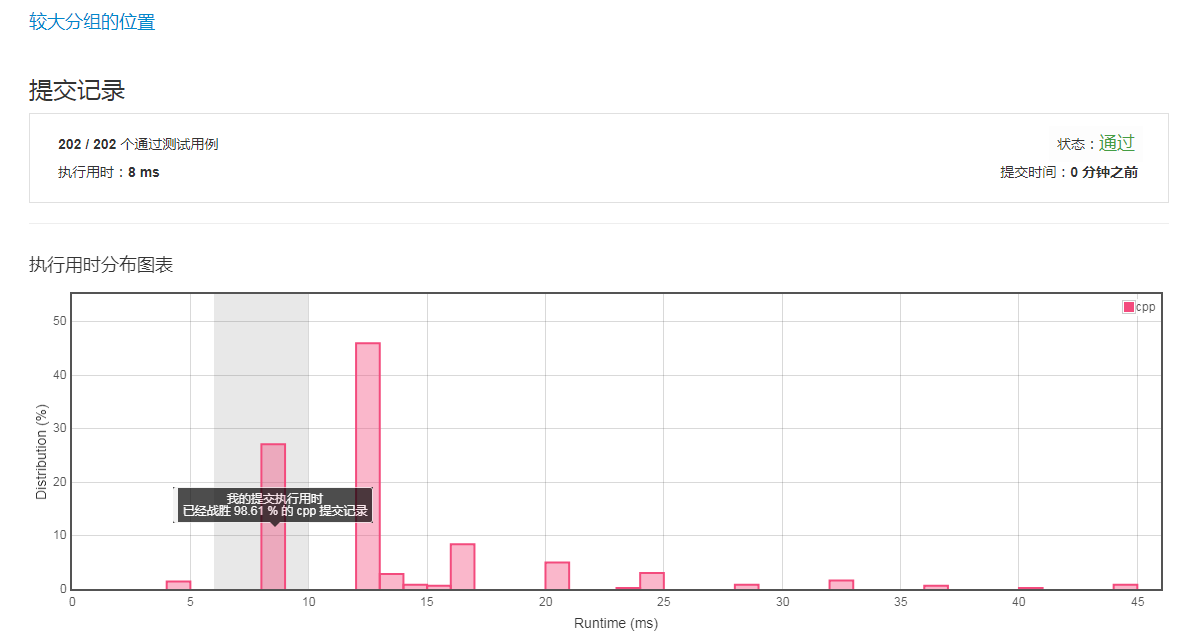## 十、 Remove Element

### 题目如下：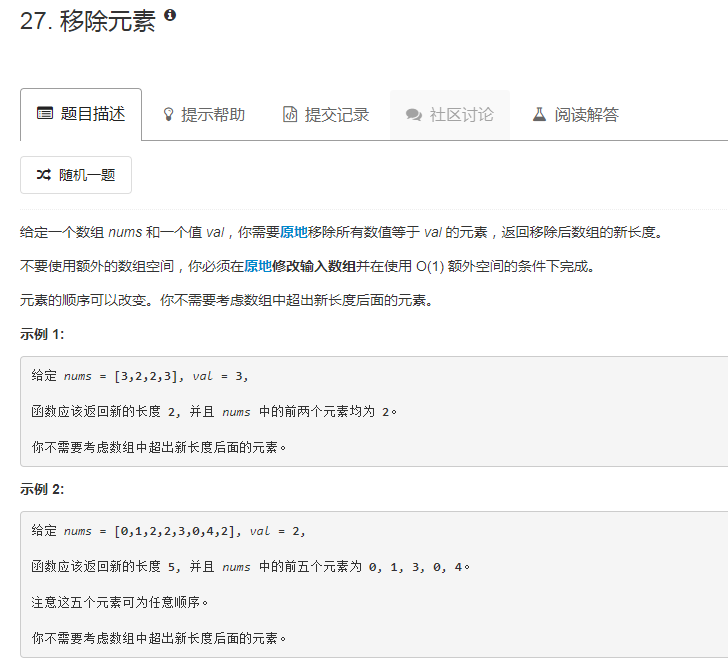### 解题如下：

class Solution {

public:

int removeElement(vector<int>& nums, int val)
{
int  slow_ptr =0;

for (int fast_ptr = 0; fast_ptr < nums.size();fast_ptr++ )
{
if(nums[fast_ptr] != val)
{
nums[slow_ptr] = nums[fast_ptr];
slow_ptr ++;
}
}

return slow_ptr;
}
};

### 提交结果：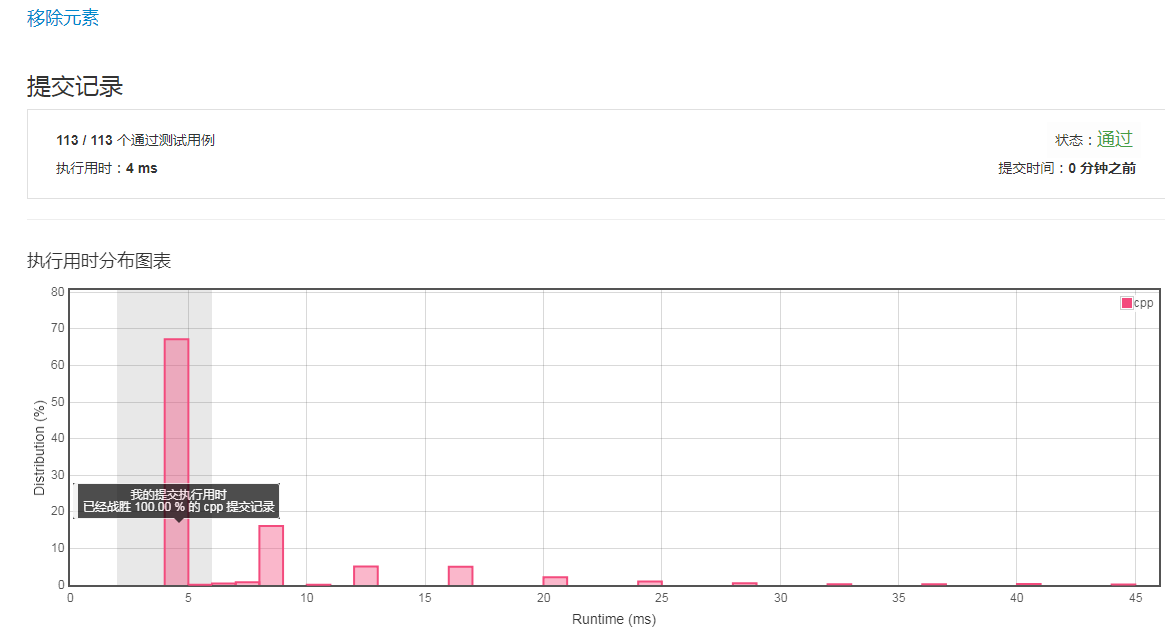## 十一、Remove Duplicates from Sorted List

### 题目如下：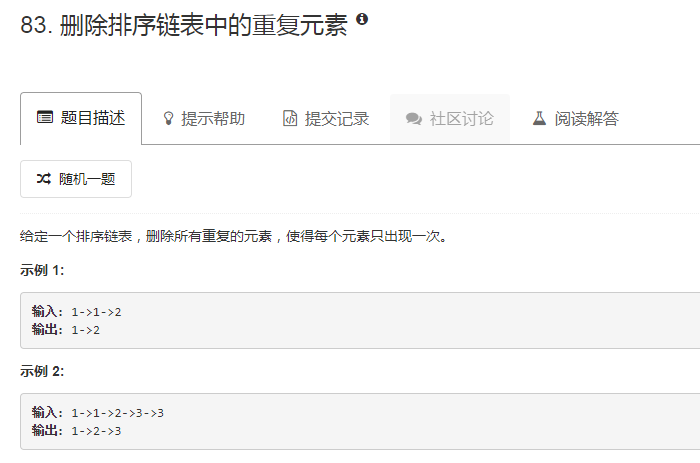### 解答如下：

/**
* struct ListNode {
*     int val;
*     ListNode *next;
*     ListNode(int x) : val(x), next(NULL) {}   //默认构造函数
* };
*/

class Solution {

public:

{

while(fast_ptr != NULL)
{
if (fast_ptr->val != low_ptr->val)
{
low_ptr->next = fast_ptr;
low_ptr = low_ptr->next;
}

else      // 删除重复的节点
{
ListNode* tem_ptr = fast_ptr;
delete tem_ptr;
}

fast_ptr = fast_ptr->next;
}

low_ptr ->next = NULL;

}
};

### 提交结果：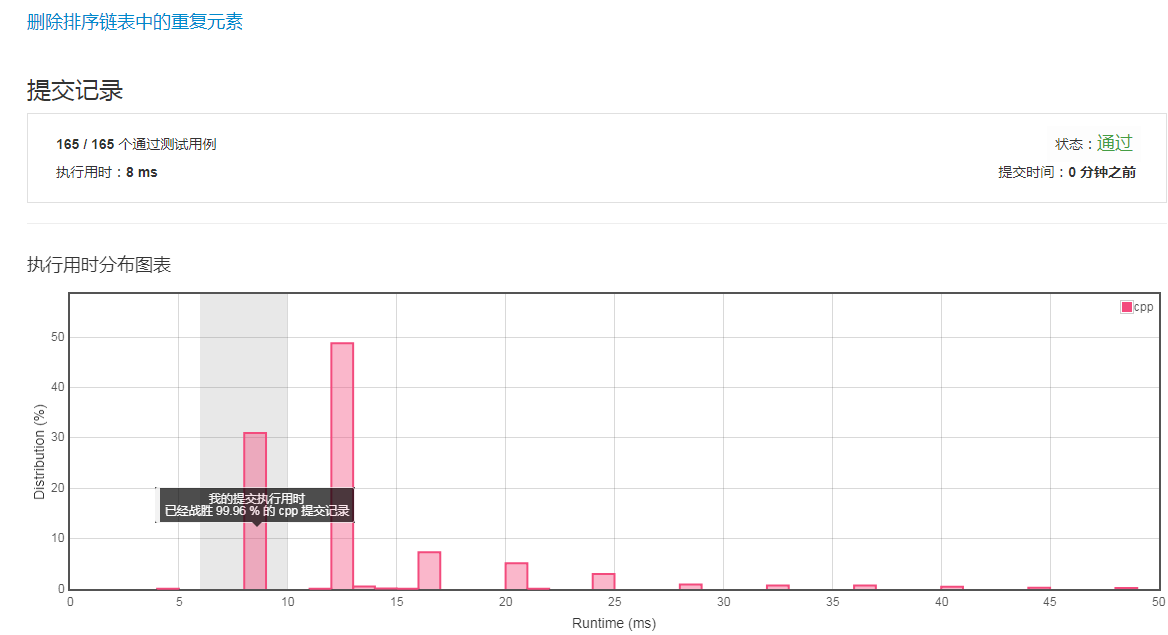### 题目如下：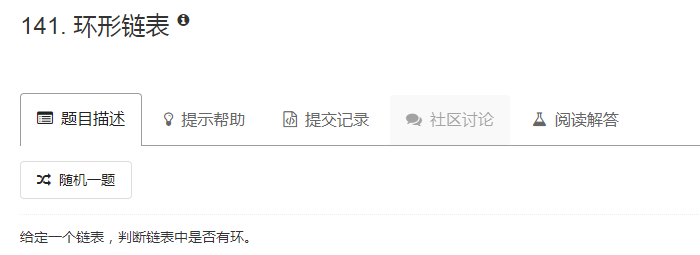### 解答如下：

/**
* struct ListNode {
*     int val;
*     ListNode *next;
*     ListNode(int x) : val(x), next(NULL) {}
* };
*/

class Solution {

public:

{

while (fast_ptr !=NULL && fast_ptr->next !=NULL)
{
slow_ptr = slow_ptr->next;
fast_ptr = fast_ptr->next->next;

if (slow_ptr == fast_ptr)
return true;
}

return false;
}
};

### 提交结果：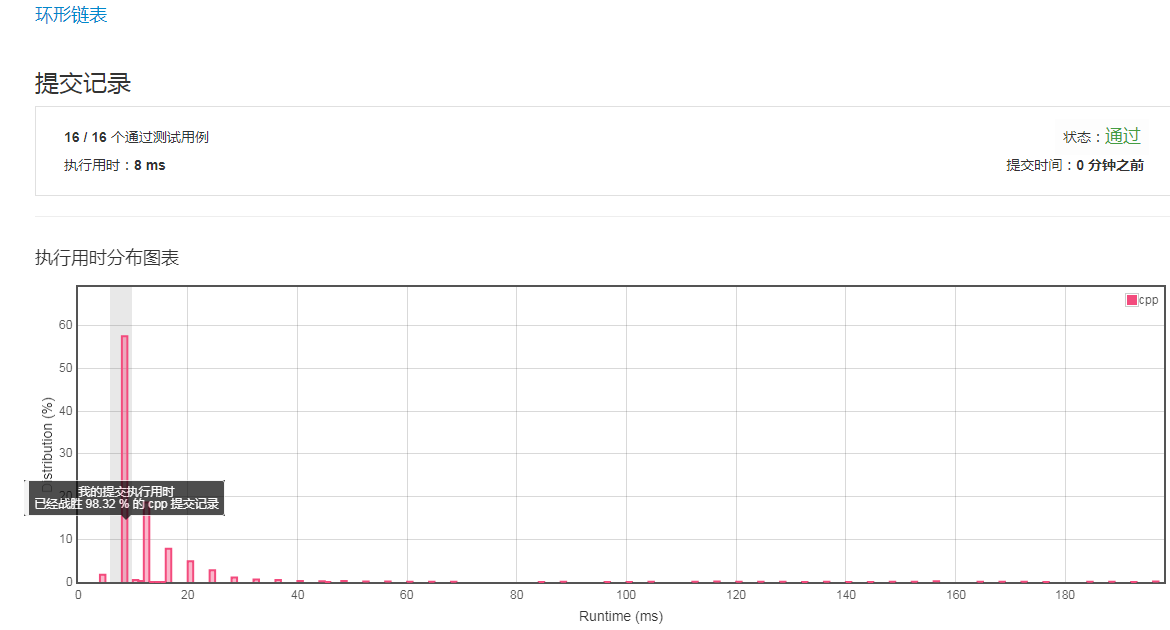## 十三、亲密字符串

###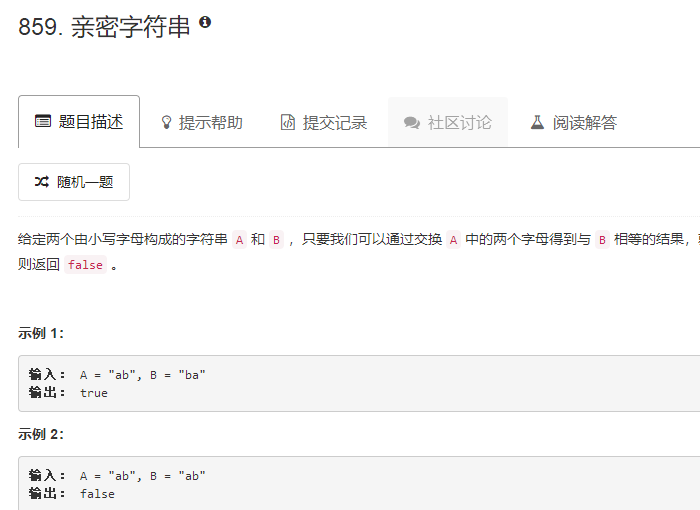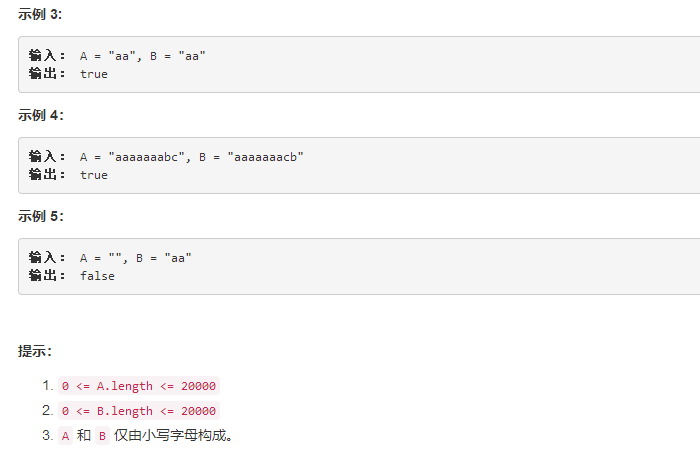### 解答如下：

class Solution {

public:

bool buddyStrings(string A, string B)
{

// 首先考虑空字符串的情况，只要有空字符串，就绝不是亲密字符串
if(A.empty() || B.empty())
{
return false;
}

// 考虑两个字符串的长度是否相等
if (A.size() != B.size())
return false;

// 考虑两个字符串对应字符全部相同的情况
// （1）例如：A = "abcdfhh", B = "abcdfhh"
// 两个字符串完全相同，因为A中有两个相同的字符，
// 因此我们可以通过交换这两个相同字符（hh）来达到字符串匹配；
// （2）例如：A = "abcdf", B = "abcdf"
// 即使两个字符串完全相同，但是不管调换，调换两个字符顺序后都无法匹配。
// 结论：相同字符串中，如果存在两个或以上的相同字符，则这两个字符串必是亲密字符串。
if (A.compare(B) ==0 )
{
sort(A.begin(), A.end());
for (int n = 0; n < A.size() - 1; n++)
{
if (A[n] == A[n + 1])
{
return true;
}
}
return false;
}

// 考虑两个字符串中有对应字符不相同的情况
vector<int> res;
for (int i = 0;i<A.size();++i)
{
if (A[i] != B[i])
res.push_back(A[i] - B[i]) ;
}

if (res.size() != 2)
return false;
else
{
if (res == -res)
return true;
else
return false;
}

}
};

## 十四、柠檬水找零

### 题目如下：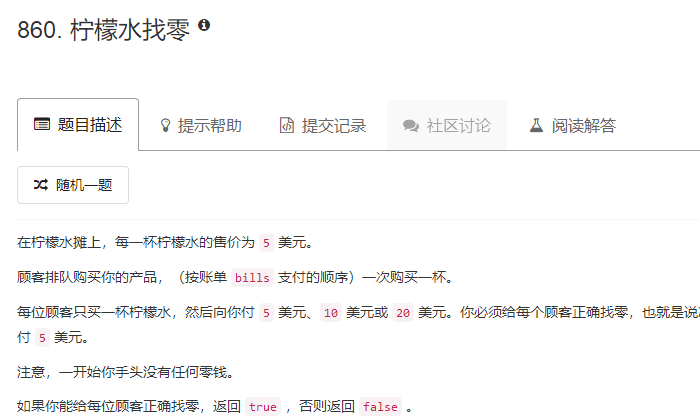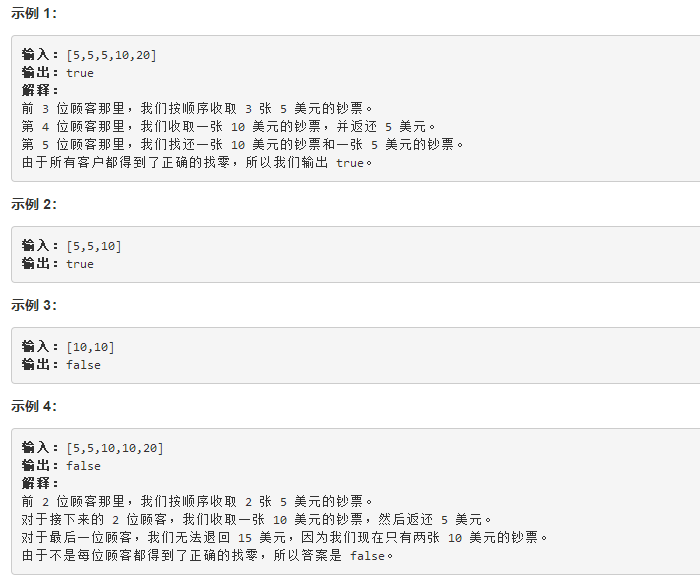### 解法（一）:

class Solution {

public:

{

int num_5 =0,num_10 =0,num_20=0;

for (int i=0;i<bills.size();++i)
{
switch(bills[i])
{
case 5:num_5++;
break;
case 10:num_10++;
break;
case 20:num_20++;
break;
default:break;
}

if ( num_5 < num_10+num_20 || (num_5-num_10-num_20)*5+num_10*10 < 10 * num_20 )
return false;
// 10元和20元找零都需要一张5元的，因此，当5元的总数少于其余两个之和，则必定无法找零。
// 当num_5 >= num_10+num_20时，需要进一步检查，是否能够找零所有20元的。
}

return true;
}
};

### 提交结果：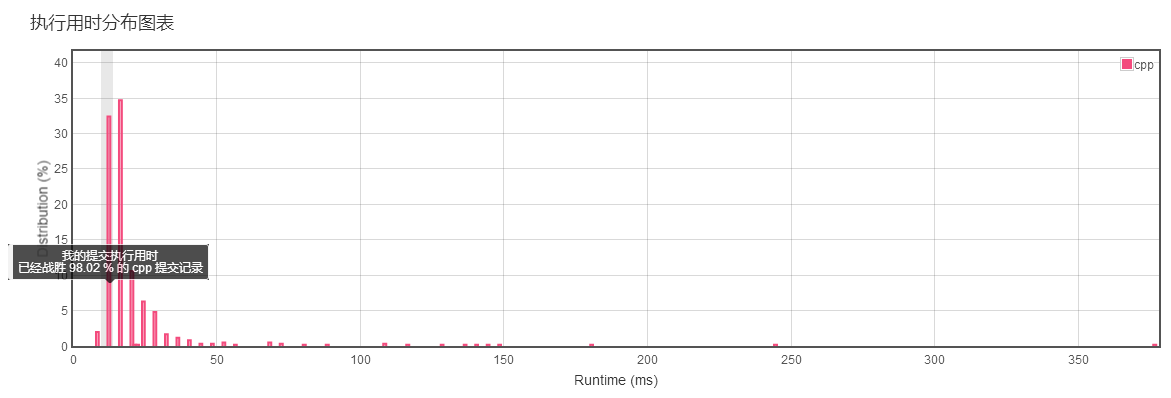### 解法（二）

class Solution {

public:

{

int num_5=0,num_10=0;

for (int i=0;i<bills.size();++i)
{
if(bills[i] == 5)
num_5++;
else if (bills[i] == 10)
{
num_5--;
num_10++;
}
else
{
if (num_10 > 0)
{
num_10--;
num_5--;
}
else
{
num_5 = num_5 - 3;
}
}

if (num_5 < 0)
return false;
}
return true;
}
};

## 十五、转置矩阵（867）

### 题目如下：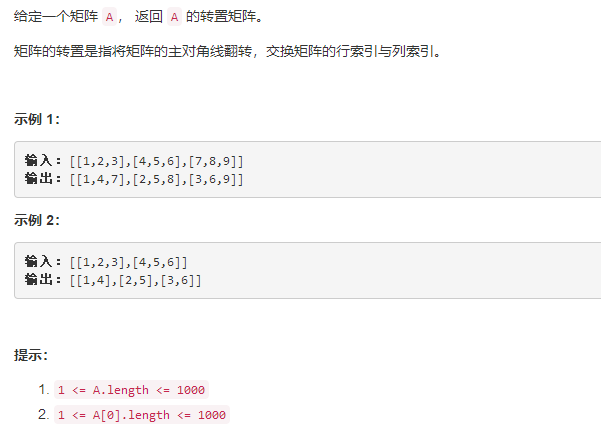### C++解答：

class Solution {

public:

vector<vector<int>> transpose(vector<vector<int>>& A)
{
vector<vector<int>> res;
int len = A.size();
for (int i=0;i<len;i++)
{
vector<int> temp;
for (int j=0;j<A.size();j++)
{
temp.push_back(A[j][i]);
}
res.push_back(temp);
}
return res;
}
};

## 十六、单调数列（896）

### 题目如下：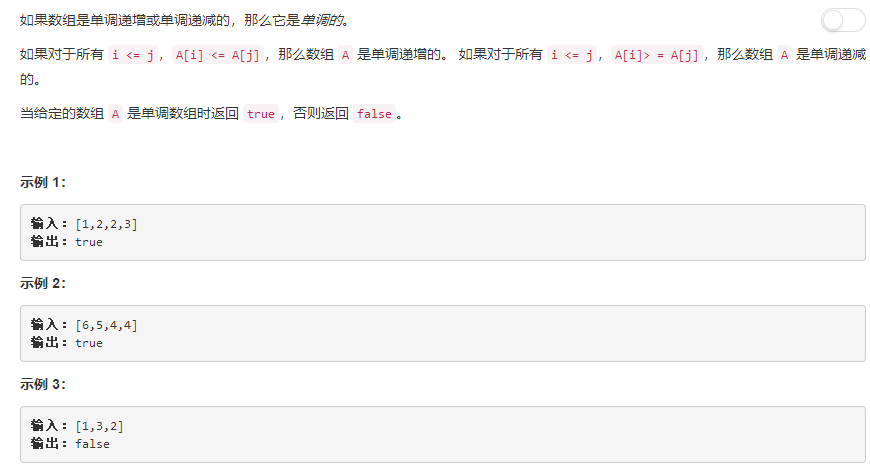### 解法（一）：

class Solution {

public:

bool isMonotonic(vector<int>& A)
{
int len = A.size();
if (A == A[len-1])
{
for (int i=1;i<len-1;i++)
if (A[i] != A)
return false;
return true;
}
else if (A > A[len-1])
{
for (int i=0;i<len-1;i++)
if (A[i] < A[i+1])
return false;
return true;
}
else
{
for (int i=0;i<len-1;i++)
if (A[i] > A[i+1])
return false;
return true;
}
}
};

### 提交结果：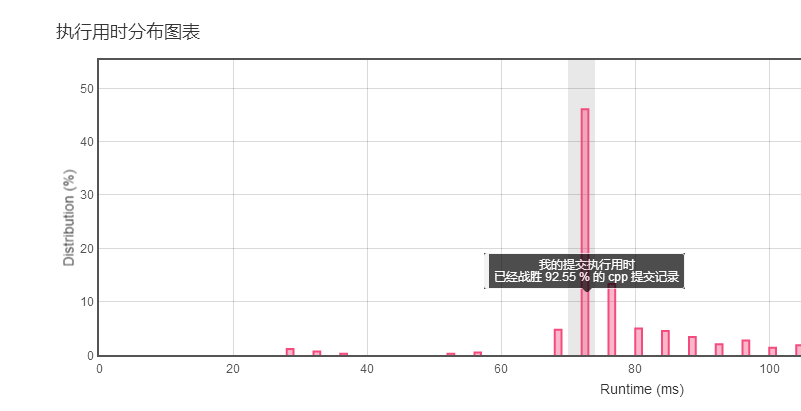### 解法（二）：

class Solution {

public:

bool isMonotonic(vector<int>& A)
{

bool inc = true, dec = true;

int n = A.size();

for(int i =0;i<n-1;i++)
{

if (A[i] > A[i+1])
inc = false;

if (A[i] < A[i+1])
dec = false;

}

return inc || dec;

}

};


### 提交结果：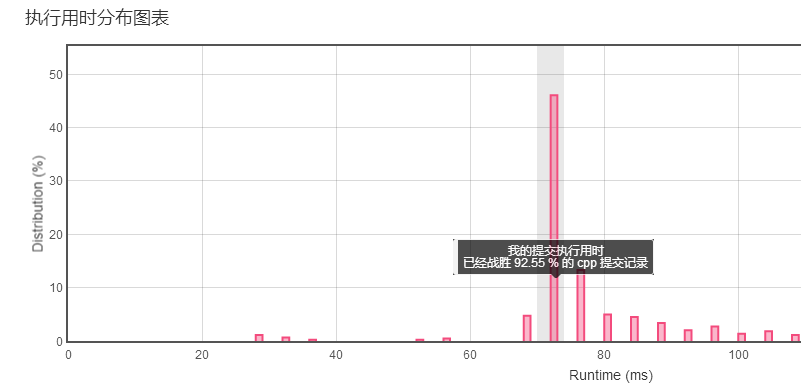## 十七、

未完待续，持续更新...

07-2104-09
08-141万+
12-03
01-26
06-29
10-05
01-14